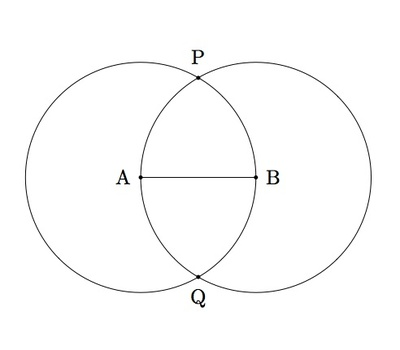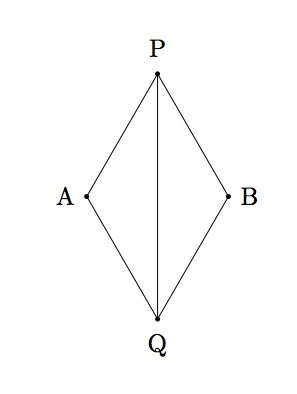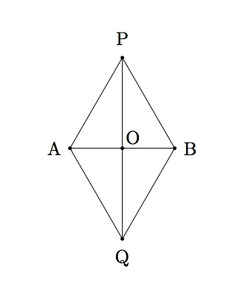# Construction of perpendicular bisector

Alignments to Content Standards: G-CO.D.12

Let $A$ and $B$ be two distinct points in the plane and $\overline{AB}$ the segment joining them. The goal of this problem is to construct the perpendicular bisector of segment $\overline{AB}$.

Draw circles with radius $|AB|$ centered at $A$ and $B$ respectively as pictured below:The two points of intersection of these circles are labelled $P$ and $Q$. Show that line $\overleftrightarrow{PQ}$ is the perpendicular bisector of $\overline{AB}$.

## IM Commentary

The construction of the perpendicular bisector of a line segment is one of the most common in plane geometry and it is undertaken here. In addition to giving students a chance to work with straightedge and compass, the problem uses triangle congruence both to show that the constructed line is perpendicular to $AB$ and to show that it bisects $AB$.

This problem assumes that the two circles intersect in points $A$ and $B$ as pictured. If this subject comes up, the teacher may wish to make explicit that the picture may be used as justification of this fact. Providing a more rigorous mathematical explanation goes beyond the scope of the high school standards.

A second solution is provided emphasizing rigid motions. The argument has two steps. First, it is possible to show that $\overleftrightarrow{AB}$ and $\overleftrightarrow{PQ}$ are perpendicular by showing that the reflection of the plane over $\overleftrightarrow{AB}$ interchanges $P$ and $Q$. Once these lines are perpendicular, showing that $\overleftrightarrow{AB}$ bisects $\overline{PQ}$ can also be shown by considering a different reflection.

This task is mainly intended for instructional purposes. The first solution is a nice application of congruent triangles but is a multi-step argument. The second solution provides an opportunity for teachers to bring in the notion of rigid motions which is behind the congruence results used in the first solution. It is important for students to see results established both in the traditional way and explicitly using rigid transformations. The construction of the perpendicular bisector is a central, foundational result and so seeing this done in both ways is vital for developing a good intuition for rigid motions.

This task includes an experimental GeoGebra worksheet, with the intent that instructors might use it to more interactively demonstrate the relevant content material. The file should be considered a draft version, and feedback on it in the comment section is highly encouraged, both in terms of suggestions for improvement and for ideas on using it effectively. The file can be run via the free online application GeoGebra, or run locally if GeoGebra has been installed on a computer.

This task walks the student through two different proofs. Any time a red dot appears, it can be clicked or moved along the circle by dragging. In the second proof, the two dots are defined as reflections of each other over the line. This is more of an illustration that requires the teacher to explain the concept. If you drag the left point, it moves the other one to show the reflection.

## Attached Resources

• 966 GeoGebra File
• ## Solutions

Solution: Congruent triangles

Notice first that triangles $PAQ$ and $PBQ$ pictured below are congruent.One way to show that triangles $PAQ$ and $PBQ$ are congruent is with SSS. These two triangles share side $\overline{PQ}$. The sides $\overline{AQ}$, $\overline{AP}$, $\overline{BQ}$, and $\overline{BP}$ are radii of circles sharing the same radius, namely $|AB|$. So all four of these line segments are congruent and triangles $PAQ$ and $PBQ$ are congruent by SSS.

We now add segment $\overline{AB}$ to the above picture, labeling the point of intersection of $\overline{PQ}$ and $\overline{AB}$ as $O$.We next show that triangles $AQO$ and $BQO$ are congruent. We know that angle $OQA$ is congruent to angle $OQB$ because these are the same angles as $AQP$and $BQP$ which are corresponding parts of congruent triangles $PAQ$ and $PBQ$. We also know that $\overline{AQ}$ is congruent to side $\overline{BQ}$ as these are also corresponding parts of congruent triangles $PAQ$ and $PBQ$. Side $\overline{OQ}$ is congruent to side $\overline{OQ}$ and so, by SAS, triangle $AQO$ is congruent to triangle $BQO$.

From the triangle congruence between triangles $AQO$ and $BQO$ we can now deduce that line $\overleftrightarrow{PQ}$ is the perpendicular bisector of segment $\overline{AB}$. Since side $\overline{AO}$ is congruent to side $\overline{BO}$ this means that line $\overleftrightarrow{PQ}$ bisects segment $\overline{AB}$. Since angle $AOQ$ is congruent to angle $BOQ$ and these two angles together form a line, this means that they are each $90$ degree angles and so line $\overleftrightarrow{PQ}$ is perpendicular to segment $\overline{AB}$. So line $\overleftrightarrow{PQ}$ is the perpendicular bisector of segment $\overline{AB}$.

Solution: Reflections

For this solution, we use the picture below which shows line $\overleftrightarrow{AB}$:Let $C_1$ denote the circle of radius $|AB|$ with center $A$ and $C_2$ the circle of radius $|AB|$ with center $B$. Consider the reflection of the plane, which we will denote by $r$, about line $\overleftrightarrow{AB}$. This line passes through the centers $A$ and $B$ of the two circles and so $r(A) = A$ and $r(B) = B$. Since $r$ preserves distances, $|Ar(x)| = |AB|$ if and only if $|Ax| = |AB|$ and similarly $|r(x)B| = |AB|$ if and only if $|xB| = |AB|$. This means that $r(C_1) = C_1$ and $r(C_2) = C_2$. In particular $r(C_1)$ and $r(C_2)$ meet in the same two points, $P$ and $Q$, as $C_1$ and $C_2$. Since $r$ exchanges the points above $\overleftrightarrow{AB}$ with the points below $\overleftrightarrow{AB}$ we conclude that $r(P) = Q$ and $r(Q) = P$. By definition of reflections, we conclude that $\overleftrightarrow{AB}$ is the perpendicular bisector of $\overline{PQ}$. Hence $\overleftrightarrow{PQ}$ is perpendicular to $\overline{AB}$ and it remains to show that it also bisects $\overline{AB}$.

Since $r(\overline{AP}) = \overline{AQ}$ and $r$ preserves distances we know that $|AP| = |AQ|$. Since $r(\overline{BP}) = \overline{BQ}$ and $r$ preserves distances we know that $|BP| = |BQ|$. Segement $\overline{PQ}$ is congruent to itself so by SSS we know that $\triangle APQ$ is congruent to $\triangle BPQ$. In particular $m(\angle APQ) = m(\angle BPQ)$. Let $s$ denote the reflection of the plane about line $\overleftrightarrow{PQ}$. Since $s$ preserves angles we conclude that $s(\overrightarrow{PA}) = s(\overrightarrow{PB}))$. Since $s$ preserves distances and $|PA| = |PB|$ it follows that $s(A) = B$. The definition of reflection about $\overleftrightarrow{PQ}$ tells us that $\overleftrightarrow{PQ}$ is the perpendicular bisector of $\overline{AB}$ as desired.